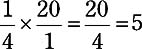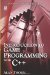# 1.10 Percentage

## 1.10 Percentage

Percentages uses the % sign. We say 25% to mean ¼ and 50% to mean ½, and 100% to mean 1 whole unit. Percent, then, describes a quantity in terms of 100 ("out of 100"), so therefore percentage can be seen to describe a ratio of a number to 100. The following demonstrate some examples of percentages:

• 25%-Is one quarter because 25: 100 (100 is four times 25, and 25 is the fourth part of 100).

• 50%-Is half because of the ratio 50: 100 (50 is half of 100, and 100 is two times 50).

• 200%-Is two times 100 because 200: 100 (200 is two times 100, and 100 is half of 200).

### 1.10.1 As Ratio

The concept of percent being a ratio to 100 can help solve problems related to percentage.

What is 300% of 10?

The ratio of 100: 100 is 1: 1; so 100% of 10 is the whole item, or 10. 200% is the ratio of 200: 100. So what number is to 10 as 200 is to 100? 200 is two times 100, and 100 is half of 200. Therefore, 10 is half of 20, and 20 is two times 10. So 20 is 200% of 10. 300% must therefore be three times 10. 300% of 10 is 30.

What is 50% of 30?

50: 100 is the ratio of 50%. 100 is two times 50, and 50 is half of 100. So what number is to 30 as 50 is to 100? We must complete a proportion. The answer is 15 because 15 is half of 30, and 30 is two times 15.

### 1.10.2 Percent as Fractions

Since percentages describe a number's ratio to 100, and since fractions represent the ratio of the numerator to the denominator, percentage can also be represented as a fraction with a denominator of 100. So 50% = 50/100, 25% = 25/100, and 75% is 75/100, etc.

Furthermore, since every percentage can be seen as a fraction over 100, it follows that every percentage can be represented easily in decimal: 25% = 25/100 = 0.25 and 75% = 75/100 = 0.75.

### 1.10.3 Percent Of

We have seen how to find a specific percent of a number by using the idea of ratio. For example, 300% of 10 is 30, 50% of 40 is 20, and 25% of 60 is 15. An arithmetical method, however, is simply the multiplication of fractions. 25% can be written as a fraction over 100 as 25/100. To find 25% of 20, reduce 25/100 to ¼:Note Another method of finding a percentage of a total value is to multiply by the decimal fraction. For example, 25% of 20 = 0.25 ×Introduction to Game Programming with C++ (Wordware Game Developers Library)
ISBN: 1598220322
EAN: 2147483647
Year: 2007
Pages: 225
Authors: Alan Thorn

Similar book on Amazon

flylib.com © 2008-2017.
If you may any questions please contact us: flylib@qtcs.net